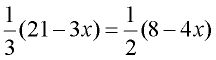Solving Linear Equations Practice Terms of Use    Contact Person: Donna RobertsDirections: Solve the following equations, for the indicated variable. Be careful! The students' choices may, or may not, be correct.

1.
Solve for x:    3x - 12 = 0Choose:
 x = 4 x = 8 x = -4 x = -8

2.
Solve for m:     2(m + 6) = 48Choose:
 m = 18 m = 21 m = 24 m = 30

3.
Solve for x:     3(2x - 1) - 10 = 8 + 5xChoose:
 -7 -3 19 21

4.
Solve for x:    8x + 9 - 3x = 8 + 5x + 1Choose:
 x = 9 x = 5 No Real numbers work. All Real numbers work.

5.
Solve for a:    8a - (4a + 32) = 16Choose:
 a = 12 a = -4 a = 8 a = 4

6.
Solve for x:    5(2x - 1) = 4(3x - 2)Choose:
 x = 13/2 x = 3/2 x = 1/2 x = 3

7.
Solve for m:     0.02m + 0.08(8 - m) = 1.78Choose:
 m = 41 m = -1.98 m = 19 m = -19

8.
Solve for x:    4(x + 5) = 3(x - 2) - 2(x + 2)Choose:
 x = -1 x = -4 x = -6 x = -10

9.
Solve for x:     6x - [2x + 3(x + 1)] = x + 20Choose:
 x = 17 x = 21 No Real numbers work. All Real numbers work.

10.
Solve for x:Choose:
 x = -3 x = 3 x = 11 x = -11